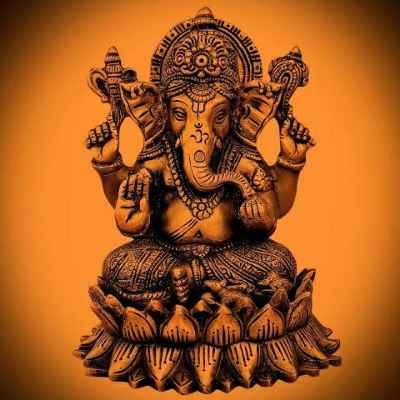Forums

# Theory of demand

When demand and quantity demand is in same direction then elasticity of demand is positive or negativeRupesh Virat

CA Foundation

865

16-Jun-20

79

Query is not clear. if you are referring to this based on a book, please share a screenshot. will be helpfulSriram Somayajula

16-Jun-20

Query is not clear. if you are referring to this based on a book, please share a screenshot. will be helpful

If pirce increases by 50% if demand also increases by 50% then price elasticity of demand is -1or1 explainRupesh Virat

CA Foundation

865

16-Jun-20

When the percentage change in quantity demanded is equal to that in price, price elasticity of demand is unit (or unitary) elastic (Ed = â??1) .CA Inter

2K+

16-Jun-20

When the percentage change in quantity demanded is equal to that in price, price elasticity of demand is unit (or unitary) elastic (Ed = â??1) .

But price and demand increasing in same direction so we should take +1or -1Rupesh Virat

CA Foundation

865

17-Jun-20

When the price and the demand increase/decrease, in same proportion (say both increase/decrease by 10%), then the elasticity of demand will be +1. in different proportions (say both increase or decrease - demand by 10% and price by 4% or vice-versa), then the elasticity of demand will be positive.CA Inter

2K+

17-Jun-20

Please refer pages2.38, 2.40 and 2.41 of study material. Link below https://resource.cdn.icai.org/46695bosfnd-p4-cp2-u1.pdf Price Elasticity when computed is negative, as price and demand of a product are inversely related. However elasticity is stated usually without the sign & is written as a positive number. The value of elasticity varies from 0 to infinity In your question if 50% change in price results in 50% change in demand then elasticity is Unitary i.e exactly 1 with +ve sign Elasticity is greater than one when the percentage change in quantity demanded is greater than the percentage change in price. In such a case, demand is said to be elastic. Elasticity is less than one when the percentage change in quantity demanded is less than the percentage change in price. In such a case, demand is said to be inelasticSriram Somayajula

17-Jun-20

Here demand is in same direction so which sign we should allot to it 1or-1Rupesh Virat

CA Foundation

865

19-Jun-20

Here demand and price is in same direction .so my question is if price increases by 50 percentage then if demand exeptionally increased by 50 percentage then we allot positive sign or negative signRupesh Virat

CA Foundation

865

19-Jun-20

When the price and the demand increase/decrease, in same proportion (say both increase/decrease by 10%), then the elasticity of demand will be +1. in different proportions (say both increase or decrease - demand by 10% and price by 4% or vice-versa), then the elasticity of demand will be positive.

Iam not asking about proportions. My question is if price increases demand increased expectionally then which sign we should allot to it positive or negativeRupesh Virat

CA Foundation

865

19-Jun-20

Rupesh Virat

Here demand and price is in same direction .so my question is if price increases by 50 percentage then if demand exeptionally increased by 50 percentage then we allot positive sign or negative sign

Which sign we will takeRupesh Virat

CA Foundation

865

19-Jun-20

Rupesh Virat

Which sign we will take

Price Elasticity does not have a negative sign. It is always stated as a positive value onlySriram Somayajula

19-Jun-20

Relation between price and demand is oppositeZeeyan KOKA

CA Foundation

3K+

18-Aug-20

When the percentage change in quantity demanded is equal to that in price, price elasticity of demand is unit (or unitary) elastic (Ed = â??1) .

+1,-1Vivek raj gaming

CA Foundation

3K+

05-Sep-20

Sales